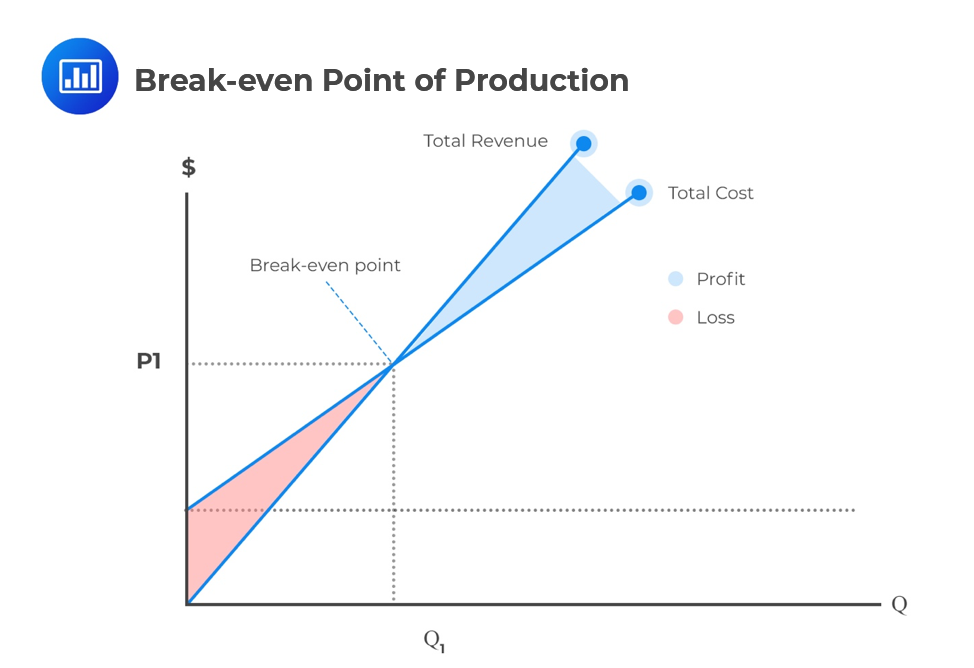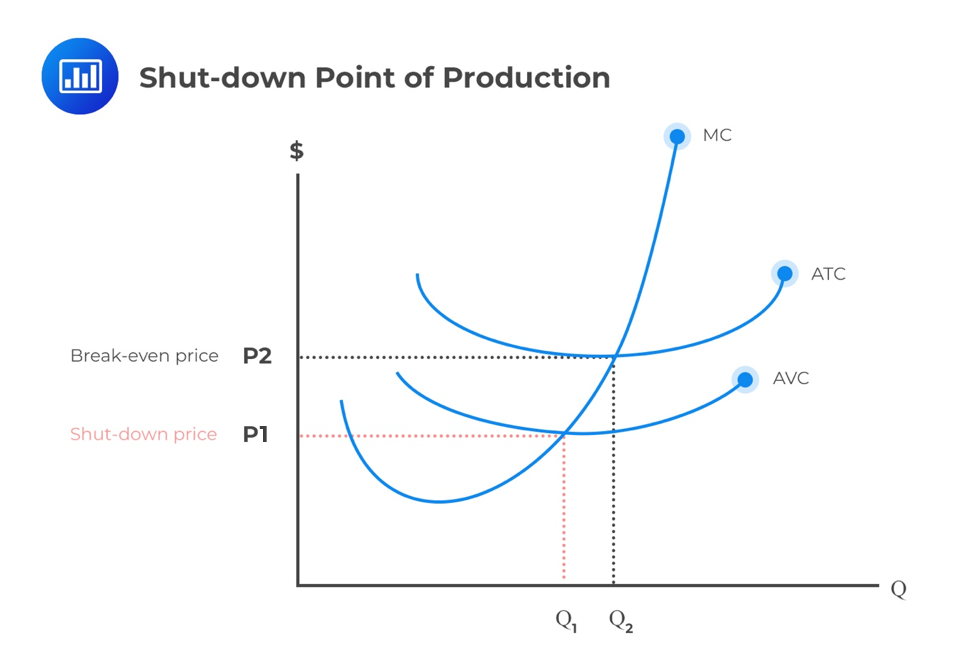Limited Time Offer: Save 10% on all 2021 and 2022 Premium Study Packages with promo code: BLOG10    Select your Premium Package »# Break-even and Shut-down Points of Production

## Break-even Point of Production

The break-even point can be defined as the production and sales levels of a given product at which the revenue generated from the sales is perfectly equal to the production cost. At this point, the company does not make any profit or loss; it breaks even.

The production cost of every product is divided into two components: the fixed cost components and the variable cost components.

### Fixed Costs

Fixed costs are those costs that remain the same regardless of the level of production of any company. A good example is the cost incurred in setting up production facilities, e.g., rent, fixed interest charges, and depreciation.

### Variable Costs

Variable costs are directly proportional to the volume produced, and examples include raw materials, wages paid, and other incurred expenses.

A company must fix its selling price above the variable costs incurred per unit of production. The difference between the selling price and the variable costs is referred to as the contribution to fixed costs and profits.

An increase in sales causes a direct increase in contribution. At the break-even point of sales, the contribution is strictly equal to the fixed cost. Therefore, companies will report losses below this point and profits above this point.

After identifying the types of production costs, it is now possible to calculate the break-even point of production because this is where you will either make a profit or a loss.If we want to determine the number of units that can be produced and sold to break even, we can use the following formula:

Break-even point of production=$$\frac{FC}{P-VC}$$

Where,

$$FC$$=Fixed cost

$$P$$=Selling Price per unit of production

$$VC$$=Variable cost per unit

Note that the denominator of the above formula is the contribution margin per unit of production.

The total fixed cost of a manufacturing company is $300,000, and the variable cost per unit produced is$150. If the selling price of one unit is $300, calculate the break-even point of production. Solution We know that: break-even point of production=$$\frac{FC}{P-VC}=\frac{300,000}{300-150}=2,000$$; and this is the number of units that must be produced and sold to break even. ## Shut-down Point of Production The shut-down point refers to the minimum price at companies that prefer shutting down their operation instead of continuing to operate. In other words, it is the minimum price and quantity for keeping operations open. The variable cost per unit (written as marginal cost, MC, on the following graph) falls with an increase in the number of units produced up to a certain point. After this point, the variable cost rises. Consequently, a U-shaped curve is realized when quantity is plotted on the x-axis against the average variable cost on the y-axis.As seen previously, the break-even point is the point where the marginal cost (MC) equals the average total cost (AT C). The shut-down point of production, on the other hand, is the price at which the marginal cost equals average variable costs (AVC). At this point, the company is better off stopping its production than keeping producing at a loss. #### Example: Shut-Down Point of Production Assume that a manufacturing company produces 1000 units and sells them at$5 each. Then the Total Revenue (TR) is 5 × 1,000=$5,000. The Average Total Cost (ATC) is$7,000 with a fixed cost (FC) of $4000 and a variable cost (VC) of$3,000 for all units. Evidently, this manufacturing company is operating at a loss of -$2000 (economic loss). In economics, we assume that the FC cannot be avoided. The company is obliged to pay it up regardless of whether it operates or not. That is, if it closes its operations, the revenue will be zero but it will still incur a$4,000 fixed cost.

If it continues its operations, it will earn a revenue of $5000, pay a variable cost of$3000 and use \$2000 to pay the fixed cost. In this instance, the company will lose less by continuing its operations. However, the company will exit the market in the long run unless prices increase because eventually, the average variable costs exceed average revenue (AR). Thus it will shut down at the point of minimum average variable cost (AVC), as seen on the graph.

### Question

The short-term shut-down point of production for a firm operating under perfect competition will most likely occur when the price per unit is equal to:

1.  marginal cost per unit.
2.  average total cost per unit.
3. average variable cost per unit.

Solution

Any firm will shut down its production when the marginal cost is less than average variable cost. We will see later that for a firm in perfect competition to maximize profit, marginal revenue must be equal to marginal cost.

Featured Study with UsCFA® Exam and FRM® Exam Prep Platform offered by AnalystPrep

Study Platform

Learn with Us

Subscribe to our newsletter and keep up with the latest and greatest tips for success
Online TutoringOur videos feature professional educators presenting in-depth explanations of all topics introduced in the curriculum.

Video LessonsSergio Torrico
2021-07-23
Excelente para el FRM 2 Escribo esta revisión en español para los hispanohablantes, soy de Bolivia, y utilicé AnalystPrep para dudas y consultas sobre mi preparación para el FRM nivel 2 (lo tomé una sola vez y aprobé muy bien), siempre tuve un soporte claro, directo y rápido, el material sale rápido cuando hay cambios en el temario de GARP, y los ejercicios y exámenes son muy útiles para practicar.diana
2021-07-17
So helpful. I have been using the videos to prepare for the CFA Level II exam. The videos signpost the reading contents, explain the concepts and provide additional context for specific concepts. The fun light-hearted analogies are also a welcome break to some very dry content. I usually watch the videos before going into more in-depth reading and they are a good way to avoid being overwhelmed by the sheer volume of content when you look at the readings.Kriti Dhawan
2021-07-16
A great curriculum provider. James sir explains the concept so well that rather than memorising it, you tend to intuitively understand and absorb them. Thank you ! Grateful I saw this at the right time for my CFA prep.nikhil kumar
2021-06-28
Very well explained and gives a great insight about topics in a very short time. Glad to have found Professor Forjan's lectures.Marwan
2021-06-22
Great support throughout the course by the team, did not feel neglectedBenjamin anonymous
2021-05-10
I loved using AnalystPrep for FRM. QBank is huge, videos are great. Would recommend to a friendDaniel Glyn
2021-03-24
I have finished my FRM1 thanks to AnalystPrep. And now using AnalystPrep for my FRM2 preparation. Professor Forjan is brilliant. He gives such good explanations and analogies. And more than anything makes learning fun. A big thank you to Analystprep and Professor Forjan. 5 stars all the way!michael walshe
2021-03-18
Professor James' videos are excellent for understanding the underlying theories behind financial engineering / financial analysis. The AnalystPrep videos were better than any of the others that I searched through on YouTube for providing a clear explanation of some concepts, such as Portfolio theory, CAPM, and Arbitrage Pricing theory. Watching these cleared up many of the unclarities I had in my head. Highly recommended.